Next: Lagrange points Up: Three-body problem Previous: Tisserand criterion

# Co-rotating frame

Let us transform to a non-inertial frame of reference rotating with angular velocity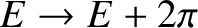about an axis normal to the orbital plane of masses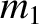and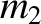, and passing through their center of mass. It follows that massesandappear stationary in this new reference frame. Let us define a Cartesian coordinate system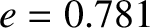in the rotating frame of reference that is such that massesandalways lie on the-axis, and the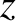-axis is parallel to the previously defined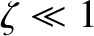-axis. It follows that massesandhave the fixed position vectors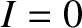and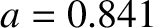in our new coordinate system. Finally, let the position vector of mass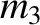be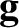. (See Figure 9.5.)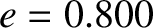According to Section 6.2, the equation of motion of massin the rotating reference frame takes the form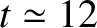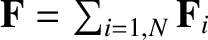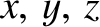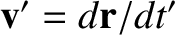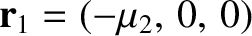(9.21)

where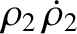, and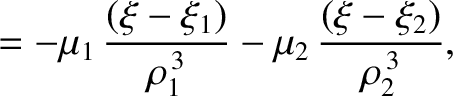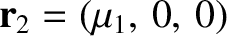(9.22)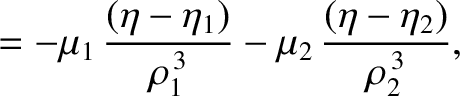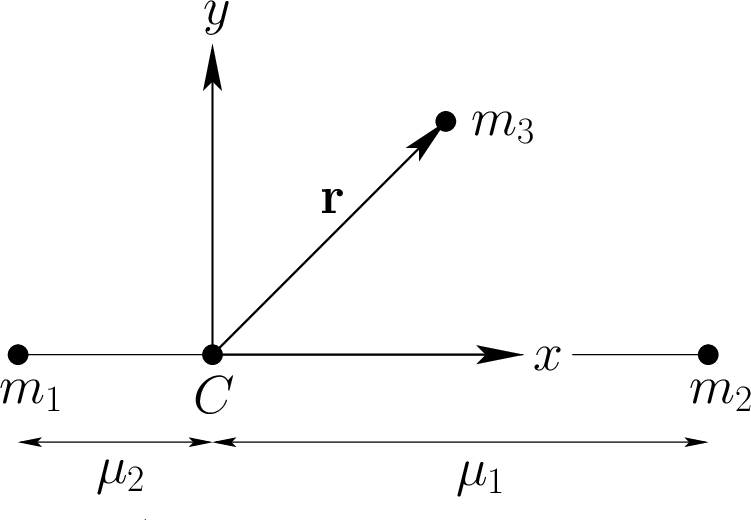(9.23)

Here, the second term on the left-hand side of Equation (9.21) is the Coriolis acceleration, whereas the final term on the right-hand side is the centrifugal acceleration. The components of Equation (9.21) reduce to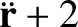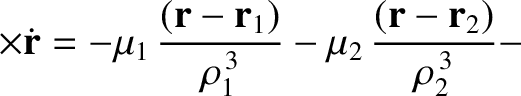(9.24)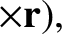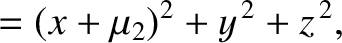(9.25) and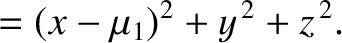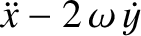(9.26)

which yield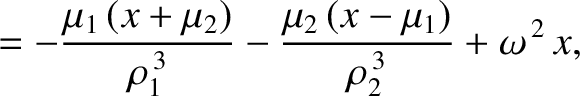(9.27)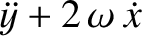(9.28) and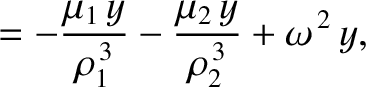(9.29)

where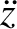(9.30)

is the sum of the gravitational and centrifugal potentials.

It follows from Equations (9.27)-(9.29) that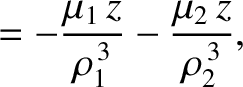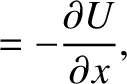(9.31)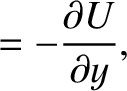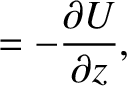(9.32) and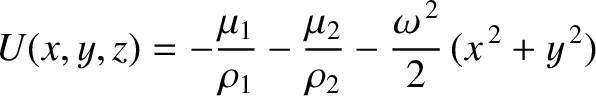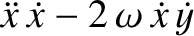(9.33)

Summing the preceding three equations, we obtain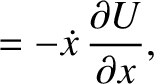(9.34)

In other words,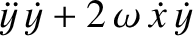(9.35)

is a constant of the motion, where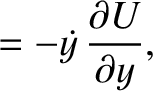. In fact,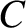is the Jacobi integral introduced in Section 9.3 [it is easily demonstrated that Equations (9.10) and (9.35) are identical; see Exercise 4]. Note, finally, that the massis restricted to regions in which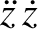(9.36)

becauseis a positive definite quantity.Next: Lagrange points Up: Three-body problem Previous: Tisserand criterion
Richard Fitzpatrick 2016-03-31